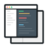# PySpark Tutorial - Learn to use Apache Spark with Python

##### PySpark Project-Get a handle on using Python with Spark through this hands-on data processing spark python tutorial.#### Videos

Each project comes with 2-5 hours of micro-videos explaining the solution.#### Code & Dataset## What will you learn

Overview of the project, its motive and expected output
What is Pyspark
Spark as a Bigdata Cluster Computing framework
Installing Anaconda and Spark
Interaction with Spark Shell using Python API
Understanding Transformation and Actions using Spark
Establishing Spark Environment and creating a handshake function between Python and Spark
What is Resilient Distributed Data-RDD and performing RDD operation
Creating RDD partitions and Instances
Performing Basic Descriptive Statistics using PySpark
Performing Basic Statistical Test in PySpark
Understanding Linear Relation and calculating Correlation
Performing the Chi-Squared test for non-linear relation
Importing necessary library for implementing model on datapoints
Using Map and lambda function to read a dataset
Applying the Logistic Regression model for training and making final predictions

## Project Description

This series of PySpark project will look at installing Apache Spark on the cluster and explore various data analysis tasks using PySpark for various big data and data science applications.

This video PySpark tutorial explains various transformations and actions that can be performed using PySpark with multiple examples.

## Similar Projects

#### Implement Back-Propagation Algorithm for Classification Problems

In this machine learning project, we will implement Back-propagation Algorithm from scratch for classification problems.

#### Customer Churn Prediction Analysis using Ensemble Techniques

In this machine learning churn project, we implement a churn prediction model in python using ensemble techniques.

#### Spark Project-Analysis and Visualization on Yelp Dataset

The goal of this Spark project is to analyze business reviews from Yelp dataset and ingest the final output of data processing in Elastic Search.Also, use the visualisation tool in the ELK stack to visualize various kinds of ad-hoc reports from the data.

## Curriculum For This Mini Project

Overview of Project
00m
What is PySpark
01m
Install PySpark
05m
Handshake between Python and Spark
12m
RDD - Resilient Distributed Data
03m
RDD operations
07m
Basic Statistics using PySpark
03m
Recap
02m
Basic Statistical Test
06m
Calculate Correlation
02m
Chi Squared Test
03m
Implement Machine Learning
09m
Logistic Regression Model
11m